Home | hot pepper quiz creator |
hot pepper Parameters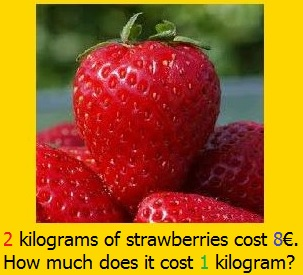You have You made a question that uses the numbers 2 8 and 1. The pupil after some training, remembers that 'the strawberry' comes with 4 (8 / 2) and disregards the way it calculated the first (maybe) time. You want In the Test (where the grades count) the same question to appear with different red number eg 4 8 or 2.5 and where, of course, the correct answer will be 2 1 and 3.2 respectively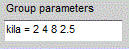How it will be done On the Groups tab, in the parameters of the group, where the question belongs, you write a parameter (eg kila) and after the equal sign = various values you want to receive. The first value is used in Training, the rest, randomly, in the Test. Caution! For decimal formation use the dot For the parameter names, use only english letters. No numbers, no dashes, no spaces. These names are case insensitive, which means that parameter kila is the same as KILA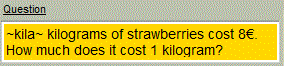In the Questions tab, you formulate the question as to the adjacent image. Notice that instead of 2 you will write ~kila~ the name of the parameter between two tildes.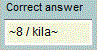For correct answer, rather than writing a neat number, you write a formula between tildes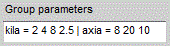If you also want to "play" with the blue value, that is the number 8 In the parameters of the group you write another parameter (eg axia) after you separate from the previous with a vertical slash. Caution! No need to receive so many values as kila, because they are independent parameters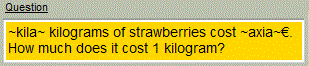You phrase the question as to the adjacent picture. Notice that instead of 8 you wrote ~axia~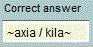For correct answer, rewrite the formula, between tildes. Caution! DO NOT use numbers that will give endless decimal digits.